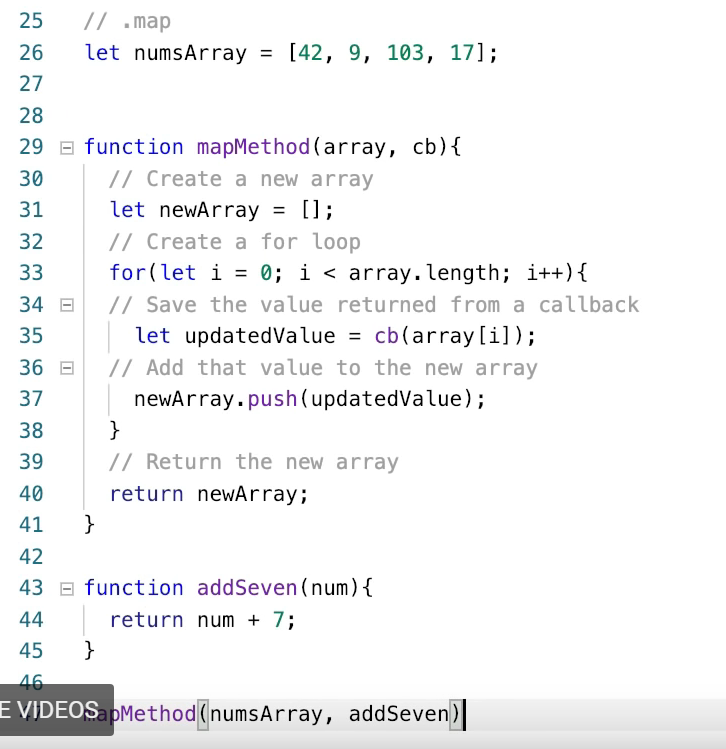← Back to all posts
JS Assignment 16: Using Callbacks in Array Methods

function exerciseOne(names){
// Exercise One: In this exercise you will be given and array called names.
// Using the forEach method and a callback as it's only argument, console log
// each of the names.
}

function exerciseTwo(cents){
// Exercise Two: In this exercise you will be given an array called 'cents'
// This array is a list of prices, but everything is in cents instead of dollars.
// Using the map method, divide every value by 100 and save it as a new array 'dollars'

return dollars;
}

This is what I have:

function exerciseOne(names){
// Exercise One: In this exercise you will be given and array called names.
// Using the forEach method and a callback as it's only argument, console log
// each of the names.
console.log(names);
}

function eachMethod(array, cb){
for(let i = 0; i < array.length; i++){
cb(array[i]);
}
}
console.log(names.forEach(exerciseOne));

function exerciseTwo(cents){
// Exercise Two: In this exercise you will be given an array called 'cents'
// This array is a list of prices, but everything is in cents instead of dollars.
// Using the map method, divide every value by 100 and save it as a new array 'dollars'
return cents / 100;
}
dollars.map(exerciseTwo);
return dollars;
}

What did I do wrong?

### Exercise One:

It ask you to console.log each individual element, not the entire array:

``````function exerciseOne(names) {
names.forEach(elem => console.log(elem));
}``````

### Exercise Two:

You are doing code that makes no sense. You have to do this:

``````function exerciseTwo(cents) {
var dollars = cents.map(elem => elem / 100);
return dollars;
}``````

If this answers your question, please check the checkmark on the left side of this message. Thanks and Good Luck!

maximussallam (5)

malvoliothegood (366)

maximussallam (2)'s questions could be homework questions so it is best not to give him/her the answers, rather point them in the right direction or give them a similar example.

patrickvmederos (1)

@vedprad1 in the youtube video and material we are given before performing this assignment it does not make any mention of using "elem =>". My question would be how would we have known to use this => to get the correct answer? Did I miss something?edit: after messing around for with it for awhile, it tells me that this is a correct answer:

function exerciseOne(names){
// Exercise One: In this exercise you will be given an array called 'names'.
// Using the forEach method and a callback as it's only argument, console log
// each of the names.

names.forEach(function (names){
console.log(names);
}

)}

malvoliothegood (366)

Study these examples: https://developer.mozilla.org/en-US/docs/Web/JavaScript/Reference/Global_Objects/Array/forEach
And:
https://developer.mozilla.org/en-US/docs/Web/JavaScript/Reference/Global_Objects/Array/map
Note that the map example can be written:

``````var array1 = [1, 4, 9, 16];

// This function doubles the input value and returns it
function doubler(x) {
return x * 2;
}
// pass the function to map
const map1 = array1.map(doubler);

console.log(map1);
// expected output: Array [2, 8, 18, 32]``````
CodeDee (1)

@malvoliothegood this helped a lot, but using your example, I am not sure why the following is the following incorrect?:

``````function exerciseTwo(cents){
// Exercise Two: In this exercise you will be given an array called 'cents'
// This array is a list of prices, but everything is in cents instead of dollars.
// Using the map method, divide every value by 100 and save it as a new array 'dollars'

function dividesbyonehundred (price){
return price / 100;
}

return dollars;
}``````
CodeDee (1)

@CodeDee Nevermind! I introduced "answer" and the assignment already returned "dollars". The following is an accepted solution:

``````function exerciseTwo(cents){
function dividesbyonehundred (price){
return price / 100;
}
let dollars = cents.map(dividesbyonehundred);
return dollars;
}``````
TyNelson (2)

For Anyone wondering this is the way they actually wanted you to do problem 1. The syntax is weird it took me like 3 days to figure out. It frustrated me and i didn't want to use the "elem=>" method because it wasn't covered in curriculum. Make sure you guys are watching your braces and parenthesis smh.. Anyways, here ya go:
function exerciseOne(names){
// Exercise One: In this exercise you will be given and array called names.
// Using the forEach method and a callback as it's only argument, console log
// each of the names.
names.forEach(function(names){
console.log(names);
}
);
}

TyNelson (2)

This was the model solution to exercise two as well:
function exerciseTwo(cents){
// Exercise Two: In this exercise you will be given an array called 'cents'
// This array is a list of prices, but everything is in cents instead of dollars.
// Using the map method, divide every value by 100 and save it as a new array 'dollars'
const dollars = cents.map(function(price){
return price/100;
})

return dollars;
}

malvoliothegood (366)

@TyNelson Here is a tip. When including code in a comment bracket it with three backticks (key is underneath the Esc key) at the start and three at the end. This will preserve formatting such as tabs. E.g.

``````function exerciseTwo(cents){
// Exercise Two: In this exercise you will be given an array called 'cents'
// This array is a list of prices, but everything is in cents instead of dollars.
// Using the map method, divide every value by 100 and save it as a new array 'dollars'
const dollars = cents.map(function(price){
return price/100;
})

Indentation is accomplished using the spaces rather than tabs. Also, single lines of code can be bookended using single backticks. E.g. `const name = "Joe";`